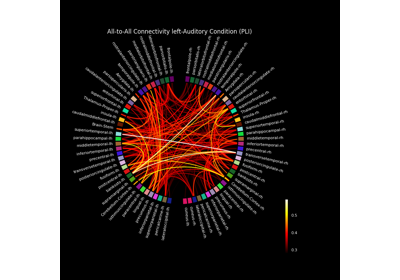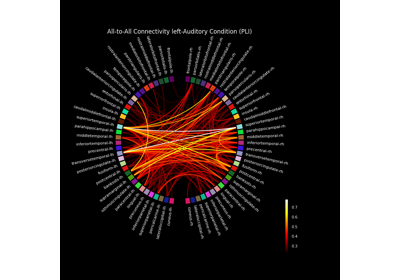# mne_connectivity.viz.plot_connectivity_circle#

mne_connectivity.viz.plot_connectivity_circle(con, node_names, indices=None, n_lines=None, node_angles=None, node_width=None, node_colors=None, facecolor='black', textcolor='white', node_edgecolor='black', linewidth=1.5, colormap='hot', vmin=None, vmax=None, colorbar=True, title=None, colorbar_size=0.2, colorbar_pos=(- 0.3, 0.1), fontsize_title=12, fontsize_names=8, fontsize_colorbar=8, padding=6.0, fig=None, subplot=111, interactive=True, node_linewidth=2.0, show=True)[source]#

Visualize connectivity as a circular graph.

Parameters

Connectivity scores. Can be a square matrix, or a 1D array. If a 1D array is provided, “indices” has to be used to define the connection indices.

node_names : `list` of `str`

Node names. The order corresponds to the order in con.

indices : `tuple` of `array` | `None`

Two arrays with indices of connections for which the connections strengths are defined in con. Only needed if con is a 1D array.

n_lines : `int` | `None`

If not None, only the n_lines strongest connections (strength=abs(con)) are drawn.

node_angles : `array`, shape (n_node_names,) | `None`

Array with node positions in degrees. If None, the nodes are equally spaced on the circle. See mne.viz.circular_layout.

node_width : `float` | `None`

Width of each node in degrees. If None, the minimum angle between any two nodes is used as the width.

node_colors : `list` of `tuple` | `list` of `str`

List with the color to use for each node. If fewer colors than nodes are provided, the colors will be repeated. Any color supported by matplotlib can be used, e.g., RGBA tuples, named colors.

facecolor : `str`

Color to use for background. See matplotlib.colors.

textcolor : `str`

Color to use for text. See matplotlib.colors.

node_edgecolor : `str`

Color to use for lines around nodes. See matplotlib.colors.

linewidth : `float`

Line width to use for connections.

colormap : `str` | instance of `matplotlib.colors.LinearSegmentedColormap`

Colormap to use for coloring the connections.

Minimum value for colormap. If None, it is determined automatically.

Maximum value for colormap. If None, it is determined automatically.

colorbar : bool

Display a colorbar or not.

title : `str`

The figure title.

colorbar_size : `float`

Size of the colorbar.

colorbar_pos : `tuple`, shape (2,)

Position of the colorbar.

fontsize_title : `int`

Font size to use for title.

fontsize_names : `int`

Font size to use for node names.

fontsize_colorbar : `int`

Font size to use for colorbar.

padding : `float`

Space to add around figure to accommodate long labels.

fig : `None` | instance of `matplotlib.figure.Figure`

The figure to use. If None, a new figure with the specified background color will be created.

subplot : `int` | `tuple`, shape (3,)

Location of the subplot when creating figures with multiple plots. E.g. 121 or (1, 2, 1) for 1 row, 2 columns, plot 1. See matplotlib.pyplot.subplot.

interactive : bool

When enabled, left-click on a node to show only connections to that node. Right-click shows all connections.

node_linewidth : `float`

Line with for nodes.

show : bool

Show figure if True.

Returns

fig : instance of `matplotlib.figure.Figure`

The figure handle.

axes : instance of `matplotlib.projections.polar.PolarAxes`

The subplot handle.

Notes

This code is based on a circle graph example by Nicolas P. Rougier

By default, `matplotlib.pyplot.savefig()` does not take `facecolor` into account when saving, even if set when a figure is generated. This can be addressed via, e.g.:

```>>> fig.savefig(fname_fig, facecolor='black')
```

If `facecolor` is not set via `matplotlib.pyplot.savefig()`, the figure labels, title, and legend may be cut off in the output figure.

## Examples using `mne_connectivity.viz.plot_connectivity_circle`#Compute mixed source space connectivity and visualize it using a circular graph#Compute source space connectivity and visualize it using a circular graph#